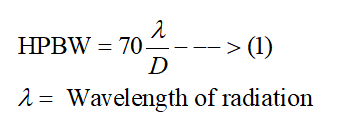# If the diameter of a parabolic reflector antenna is 1.3 m, then what will be the half power beamwidth of the antenna? Assume the wavelength of radiation to be 0.2 m.

Question
1 views

If the diameter of a parabolic reflector antenna is 1.3 m, then what will be the half power beamwidth of the antenna? Assume the wavelength of radiation to be 0.2 m.

check_circle

star
star
star
star
star
1 Rating
Step 1

The half power beamwidth (HPBW) is defined as the angular separation between the two points found on the radiation plot of the antenna with the amplitude of the two points is exactly 0.707 times the amplitude of the maximum radiation intensity.

Now, for a parabolic reflector antenna having an aperture diameter of D, the half power beamwidth (in degrees) can be expressed asStep 2

In this case, the values of the aperture diameter and the wavelength of radiation is given as

...

### Want to see the full answer?

See Solution

#### Want to see this answer and more?

Solutions are written by subject experts who are available 24/7. Questions are typically answered within 1 hour.*

See Solution
*Response times may vary by subject and question.
Tagged in

### Electrical Engineering